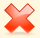## 标题：[已解決]如何更新DjangoModel之套用最大distance欄位值

2012年04月07日 星期六 21:30

vectord的值會隨著neighbours迴圈，逐漸變數字

views.py片段程式:

neighbours=[{'vector': [2, 0, 1, 0], 'label': '2'}....其他資料依此格式類推]

for nvector in neighbours:
vectord=10(屬於id=2)
"""經過一個for迴圈
vectord=4(屬於id=1)
經過一個for迴圈
vectord=3(屬於id=3)
經過一個for迴圈
vectord=8(屬於id=4)
經過一個for迴圈
vectord=2(屬於id=2)
經過一個for迴圈
vectord=2(屬於id=0.5)"""

for tid in nvector['label']:
t=Image.objects.get(pk=tid)
t.distance=vectord
t.save()

id=1 distance=4
id=2 distance=0.5
id=3 distance=3
id=4 distance=8

id=1 distance=4
id=2 distance=10
id=3 distance=3
id=4 distance=8

2012年04月08日 星期日 13:59

for nvector in neighbours:
idkey=nvector['label']
vectord=distance
t=Image.objects.get(pk=int(idkey))
t.distance=vectord
t.save()

2012年04月08日 星期日 21:38

:)如下红色区域有误，请重新填写。